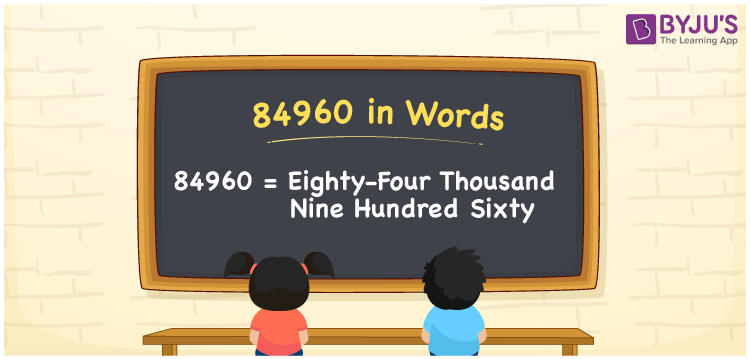# 84960 in words

84960 in words is written as Eighty Four Thousand Nine Hundred and Sixty. In 84960, 8 has a place value of ten thousand, 4 is in the place value of thousand, 9 is in the place value of hundred and 6 is in the place value of ten. The article on Place Value gives more information. The number 84960 is used in expressions that relate to money, distance, social media views, and many more. For example, “A branded smartphone costs Rupees Eighty Four Thousand Nine Hundred and Sixty.”

 84960 in words Eighty Four Thousand Nine Hundred and Sixty Eighty Four Thousand Nine Hundred and Sixty in Numbers 84960

## 84960 in English Words## How to Write 84960 in Words?

We can convert 84960 to words using a place value chart. The number 84960 has 5 digits, so let’s make a chart that shows the place value up to 5 digits.

 Ten thousand Thousands Hundreds Tens Ones 8 4 9 6 0

Thus, we can write the expanded form as:

8 × Ten thousand + 4 × Thousand + 9 × Hundred + 6 × Ten + 0 × One

= 8 × 10000 + 4 × 1000 + 9 × 100 + 6 × 10 + 0 × 1

= 84960.

= Eighty Four Thousand Nine Hundred and Sixty.

84960 is the natural number that is succeeded by 84959 and preceded by 84961.

84960 in words – Eighty Four Thousand Nine Hundred and Sixty.

Is 84960 an odd number? – No.

Is 84960 an even number? – Yes.

Is 84960 a perfect square number? – No.

Is 84960 a perfect cube number? – No.

Is 84960 a prime number? – No.

Is 84960 a composite number? – Yes.

## Solved Example

1. Write the number 84960 in expanded form

Solution: 8 x 10000 + 4 x 1000 + 9 x 100 + 6 x 10 + 0 x 1

Or Just 8 x 10000 + 4 x 1000 + 9 x 100 + 6 x 10

We can write 84960 = 80000 + 4000 + 900 + 60 + 0

= 8 x 10000 + 4 x 1000 + 9 x 100 + 6 x 10 + 0 x 1.

## Frequently Asked Questions on 84960 in words

Q1

### How to write the number 84960 in words?

84960 in words is written as Eighty Four Thousand Nine Hundred and Sixty.
Q2

### State whether True or False. 84960 is divisible by 3?

True. 84960 is divisible by 3.
Q3

### Is 84960 divisible by 10?

Yes. 84960 is divisible by 10.